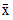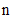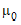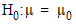# NMath User's Guide

41.2 One Sample Z-Test (.NET, C#, CSharp, VB, Visual Basic, F#)

Class determines whether a sample from a normal distribution with known standard deviation could have a given mean. For example, suppose we wish to determine whether the IQs of children from a particular school are above average, given that Wechsler IQ scores are normally distributed with a mean of 100 and standard deviation of 15. Sample scores from 9 students are 116 110 111 113 112 113 111 109 121, with a mean of 112.8.

As described Section 41.1, all hypothesis test classes provide two paths for constructing instances of that type: a parameter-based method and a data-based method. Thus, you can construct a OneSampleZTest object by explicitly specifying a sample mean (), sample size (), population mean (), and population standard deviation (), like so:

Code Example – C# z-test

```double xbar = 112.8;
```
```int n = 9;
```
```double mu0 = 100;
```
```double sigma = 15;
```
```var test = new OneSampleZTest( xbar, n, mu0, sigma );
```

Code Example – VB z-test

```Dim XBar As Double = 112.8
```
```Dim N As Integer = 9
```
```Dim Mu0 As Double = 100
```
```Dim Sigma As Double = 15
```
```Dim Test As New OneSampleZTest(XBar, N, Mu0, Sigma)
```

Or by supplying a set of sample data, and the necessary population parameters:

Code Example – C# z-test

```var data =
```
```  new DoubleVector( "[ 116 110 111 113 112 113 111 109 121 ]" );
```
```double mu0 = 100;
```
```double sigma = 15;
```
```var test = new OneSampleZTest( data, mu0, sigma );
```

Code Example – VB z-test

```Dim MYData As New DoubleVector("[ 116 110 111 113 112 113 111 109
121 ]")
```
```Dim Mu0 As Double = 100
```
```Dim Sigma As Double = 15
```
```Dim Test As New OneSampleZTest(MyData, Mu0, Sigma)
```

In this case, the sample mean and sample size are calculated from the given data.

In addition to the properties common to all hypothesis test objects (Section 41.1), a OneSampleZTest object provides the following read-only properties:

Xbar gets the sample mean.

N gets the sample size.

Mu0 gets the population mean.

Sigma gets the population standard deviation.

By default, a OneSampleZTest object performs a two-sided hypothesis test () with. In this example, we wish to test the one-sided form to the right (; that is, we wish to test whether the children in our sample have a higher than average IQ. Suppose also that we wish to set the alpha level to 0.05. Non-default test parameters can be specified at the time of construction using constructor overloads, or after construction using the provided Alpha and Type properties, like so:

Code Example – C# z-test

```test.Type = HypothesisType.Right;
```
```test.Alpha = 0.05;
```

Code Example – VB z-test

```Test.Type = HypothesisType.Right
```
```test.Alpha = 0.05
```

Once you've constructed and configured a OneSampleZTest object, you can access the test results using the provided properties, as described in Section 41.1:

Code Example – C# z-test

```Console.WriteLine( "z-statistic = " + test.Statistic );
```
```Console.WriteLine( "p-value = " + test.P );
```
```Console.WriteLine( "reject the null hypothesis? " + test.Reject);
```

Code Example – VB z-test

```Console.WriteLine("z-statistic = " & Test.Statistic)
```
```Console.WriteLine("p-value = " & Test.P)
```
```Console.WriteLine("reject the null hypothesis? " & Test.Reject)
```

The output is:

```z-statistic = 2.56
```
```p-value = 0.00523360816355578
```
```reject the null hypothesis? true
```

This indicates that we can reject the null hypotheses (). We can conclude that the children have IQs significantly above average.

Finally, remember that the ToString() method returns a formatted string representation of the complete test results:

```One Sample Z Test
```
```-----------------

```

```Sample mean = 112.8
```
```Sample size = 9
```
```Population mean = 100
```
```Population standard deviation = 15
```
```Computed Z statistic: 2.56

```

```Hypothesis type: one-sided to the right
```
```Null hypothesis: sample mean = population mean
```
```Alt hypothesis: sample mean > population mean
```
```P-value: 0.00523360816355578
```
```REJECT the null hypothesis for alpha = 0.05
```
```0.95 confidence interval: 104.575731865243 Infinity
```

Top

Top